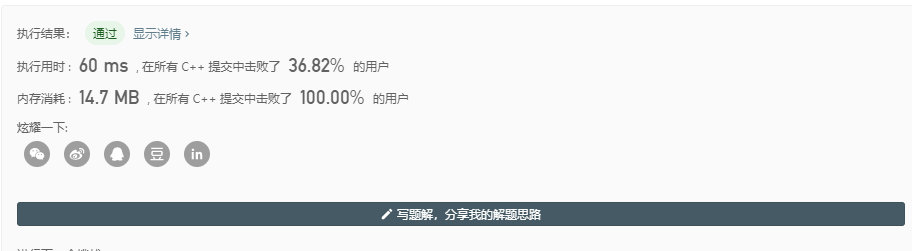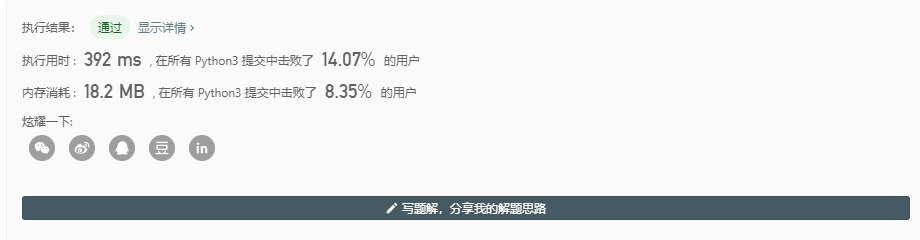# LeetCode 392.判断子序列

## 题目描述

s = “abc”, t = “ahbgdc”

s = “axc”, t = “ahbgdc”

## 解题思路

• 利用双指针方法，一个指针i来指向string s，一个指针j来指向string t，来判断string t中是否包含string s，如果找到相同的元素两个指针都像后移动一次，如果没找到相同元素j向后移动一次，判断string t包含string s的条件是：istring s的长度相同即可

### Cpp实现

class Solution {
public:
bool isSubsequence(string s, string t) {
int i = 0;
int j = 0;
while(i < s.size() && j < t.size()){
if(s[i] == t[j]){
i++;
j++;
}
else
j++;
}
return i == s.size();
}
};### Python实现

class Solution:
def isSubsequence(self, s: str, t: str) -> bool:
i = j = 0
while i < len(s) and j < len(t):
if s[i] == t[j]:
i += 1
j += 1
else:
j += 1
return i == len(s)• 时间复杂度：O(n)©️2019 CSDN 皮肤主题: 深蓝海洋 设计师: CSDN官方博客